SEARCH HOMEMath Central Quandaries & QueriesSubject: Irregular multi sided polygon Name: Graham I have an irregular polygon. I know the length of all the sides and know approximately all the internal angles. Is there a formula or table that can calculate the area for me?Hi Graham.

We have two replies for you. One from Sue

Yes there is such a formula: Heron's Formula.

Heron's formula allows you to calculate the area of a triangle if you know the lengths of all the sides of the triangle. With the information you have, you can divide the polygon into triangles whose side lengths you can calculate.

Let me show an example with an irregular pentagon: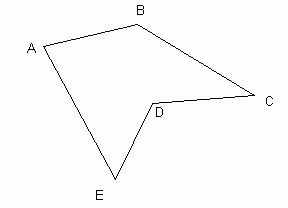Side AB is 7 units.
Side BC is 10 units.
Side CD is 7.7 units.
Side DE is 6.3 units.
Side EA is 11.2 units.
Angle A is about 76 degrees.
Angle C is about 36 degrees.

Now I'll add a couple of interior lines that divide the area into triangles: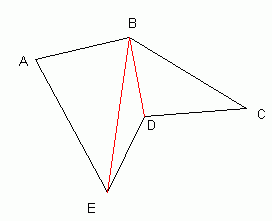In order to calculate the area of the pentagon, we can add up the areas of these three triangles we've created.

Heron's formula says:

Let the lengths of the sides of a triangle be called a, b, c.
Let the semiperimeter of the triangle (a+b+c)/2 be called s.
Then the area A of the triangle is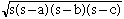.

To calculate the area of the triangles, whose sum is of course the area of the pentagon, we need to know the lengths of the red lines I added. For this, we can use the Law of Cosines:

Let the lengths of the side of a triangle be called a, b, c.
Let the angle between sides a and b be called C.
Then these quantities are related by the equation c2 = a2 + b2 - 2abCos(C).

So for our example, the Law of Cosines gives:

Side $EB = \sqrt{7^2 + 11.2^2 - (2)(7)(11.2)\cos(76^o)} = 11.68 \mbox{ units.}$
Side $DB = \sqrt{10^2 + 7.7^2 - (2)(10)(7.7)\cos(36^o)} = 5.89 \mbox{ units.}$

Note that depending on the actual polygon, you may need to also use the Law of Sines to find missing internal angles or side lengths, but in our example this isn't needed.

Now we can calculate the area of each of the three triangles using Heron's Formula:

Semiperimeter of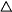ABE = (7 + 11.2 + 11.68) / 2 = 14.94 units.
Area ofABE = $\sqrt{(14.94)(14.94 - 7)(14.94 - 11.2)(14.94 - 11.68)} = 38.03 \mbox{ square units.}$

In a similar fashion the area ofEBD is 10.18 square units and Area ofBCD is 22.63 square units.

So the area of the example pentagon is $38.03 + 10.18 + 22.63 = 70.84\mbox{ square units.}$

We have many examples in our library, mostly based on people trying to calculate land measurement. Try here, here or here. The basic idea is to carve it into triangles.

Hope this helps,
Stephen La Rocque.

and a second from Chris.

The formula used by surveyors can be found in the article by Bart Braden in the COLLEGE MATH JOURNAL ("The Surveyor's Area Formula", 17:4 Sept, 1986, pages 326-337.) You can read the article on the JSTOR web page if you wish.
Start at one vertex and walk around the polygon in the counterclockwise direction. You put the first vertex at (x0, y0) = (0,0), and the next (x1, y1) = (length of first edge, 0). Subsequent points (xk, yk) are found from the previous edge using the given angle and length. (This is only practical with the help of a computer.)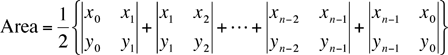For example consider the rectangle with vertices (0,0), (2,0), (2,1), (0,1) which we know has area 2.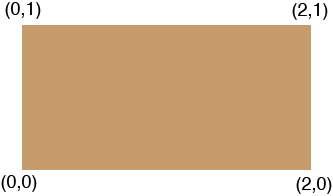The Surveyor's Area Formula gives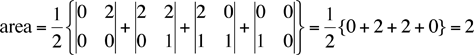There is another expression for the area in A. M. Lopshits, Computation of Areas of Oriented Figures, D. C. Heath, 1963. Translated from Russian by J. Massalski and Coley Mills, Jr.

Suppose that the side lengths of an n-sided polygon are a1, a2, ··· an and the exterior angles are φ1, φ2, ··· φn as in the diagram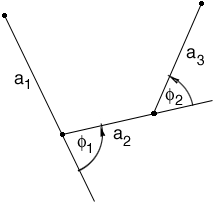Then the area of the polygon is

1/2 {a1[a2 sin 1) + a3 sin(φ1 + φ2) + ··· + an-1 sin(φ1 + φ2 + ··· + φn-2)]

+a2[a3 sin(φ2) + a4 sin(φ2 + φ3) + ··· + an-1 sin(φ2 + ··· + φn-2)]

+ ·········

+ an-2 [an-1 sin(φn-2)]}

Chris and PennyMath Central is supported by the University of Regina and The Pacific Institute for the Mathematical Sciences.